# Rubblewebs

THESE CODES ARE PROVIDED FOR AN EXAMPLE OF HOW TO USE IMAGEMAGICK WITH PHP. CARE SHOULD BE TAKEN WHEN ACCEPTING USER INPUT.

I TAKE NO RESPONSABILTY FOR ANY PROBLEMS THAT MAY OCCURE WHEN USING ANY OF THIS CODE.

IT IS UP TO YOU TO SECURE THE CODE AND VALIDATE USER INPUT.

# Noise options

## Gaussian

Add gaussian noise to an image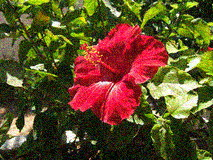```\$cmd = " \$input +noise Gaussian ";
exec("convert \$cmd gaussian.jpg");```

## Impulse

Add imulse noise to an image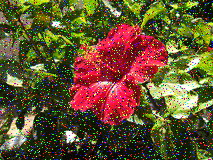```\$cmd = " \$input +noise Impulse ";
exec("convert \$cmd impulse.jpg");```

## Laplacian

Add laplacian noise to an image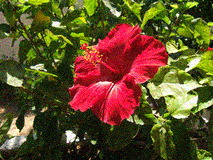```\$cmd = " \$input +noise Laplacian ";
exec("convert \$cmd laplacian.jpg");```

## Multiplicative

Add multiplicative noise to an image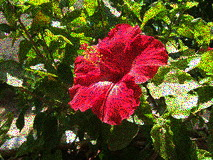```\$cmd = " \$input +noise Multiplicative ";
exec("convert \$cmd multiplicative.jpg");```

## Poisson

Add poisson noise to an image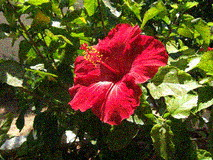```\$cmd = " \$input +noise Laplacian ";
exec("convert \$cmd poisson.jpg");```

## Random

Add random noise to an image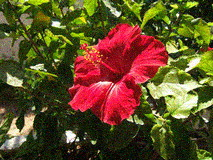```\$cmd = " \$input +noise Laplacian ";
exec("convert \$cmd random.jpg");```

## Remove noise

Remove noise from an image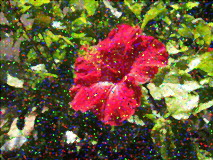```\$cmd = " impulse.jpg -noise 2 ";
exec("convert \$cmd remove.jpg");```

## Uniform

Add uniform noise to an image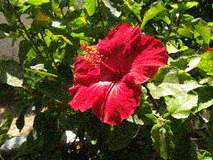```\$cmd = " \$input +noise Laplacian ";
exec("convert \$cmd uniform.jpg");```